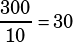there are 50 questions on a quiz given to a class of a students. Only 20% of the class answered all the questions on the quiz. Of the remaining students, half answered two-fifths of the questions and the other half answered three-fifths. What was the average number of questions answered on this quiz?

OK, so 20% of the class answered 50 questions. That leaves 80% of the class, which the question says split evenly: 40% answered 2/5 of 50 = 20 questions, and 40% answered 3/5 = 30 questions.

Let’s just assume there are 10 people in the class.

Total questions answered:Average number of questions answered: